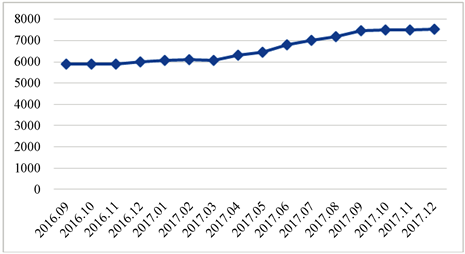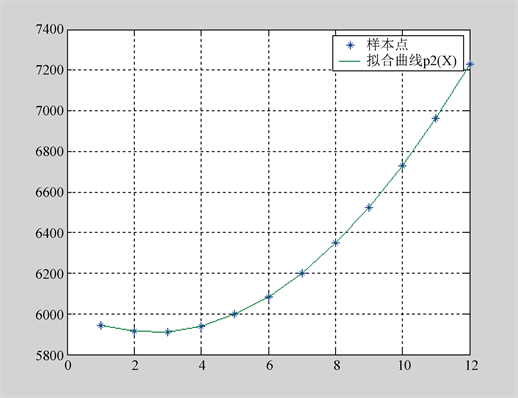﻿ 关于沈阳市沈北新区房价预测的探讨与研究

# 关于沈阳市沈北新区房价预测的探讨与研究 Discussion and Research on the Prediction of House Price in Shenbei New District of Shenyang City

Abstract: We discuss the effects to predict the trend of house price on Shenbei New Districtof Shenyang City, based on the house price data of a building in the district, respectively by the polynomial fitting method and Markov Chain method. Through a long-term follow-up statistics of the house price data on the building, repeated forecasting and empirical testing, it is concluded that the two forecasting methods are more suitable for predicting the trend of house price in the district and the effects are largely same. In addition, the method of weighted Markov Chain forecasting on house price given by Gu Ying and Xia Letian has been improved and perfected. The work we have done in this article can promote the development of the research on regional house prices.

1. 引言

2. 情况说明Table 1. The house price and its status of the A building in the moral block A in the month of September 2016 to January 2018Figure 1. A real estate in Daojie District, Shenbei New District, September 2016 to December 2017

3. 多项式回归预测

1) 根据A楼盘2016年9月~2017年8月逐月房价数据，寻找多项式拟合回归模型，并预测2017年9月房价。Figure 2. Based on building A September 2016-August 2017 Quadratic fitting Curve Image of House Price

2) 依次根据A楼盘2016年10月~2017年9月，2016年11月~2017年10月，2016年12月~2017年11月，2017年1月~2017年12月的逐月房价数据，运用1)中方法预测接下来四个月2017年10月，2017年11月，2017年12月，2018年1月的房价。

3) 说明与讨论Table 2. Example of house price forecast value, actual value and absolute deviation sumTable 3. Absolute deviation corresponding level partitioning

4. 加权马尔可夫链预测

4.1. 方法介绍

${x}_{l},l=1,2,\cdots ,n$，为连续n个度量期的房价，可用加权马尔可夫链方法预测第 $\left(n+1\right)$ 个度量期的房价，具体方法请见文  中2.3节。需要说明的是，在文  中，顾莹和夏乐天是用系统聚类–离差平方和法进行状态分类的，这种方法不是唯一的，实用中可以用其它方法进行分类。

4.1.1. 分类Table 4. A partitioning of housing price statesTable 5. A property price index and its corresponding status from September 2016 to August 2017

4.1.2. 马氏性检验

$\frac{{p}_{ij}}{{p}_{\cdot j}}=\frac{\left(\frac{{f}_{ij}}{\underset{k=1}{\overset{m}{\sum }}{f}_{kj}}\right)\left(\underset{i=1}{\overset{m}{\sum }}\underset{j=1}{\overset{m}{\sum }}{f}_{ij}\right)}{\underset{j=1}{\overset{m}{\sum }}{f}_{ij}}=\frac{{f}_{ij}\left(\underset{i=1}{\overset{m}{\sum }}\underset{j=1}{\overset{m}{\sum }}{f}_{ij}\right)}{\left(\underset{j=1}{\overset{m}{\sum }}{f}_{ij}\right)\left(\underset{k=1}{\overset{m}{\sum }}{f}_{kj}\right)}=\frac{{f}_{ij}\cdot \sigma }{{f}_{ij}+\delta }$ ,

4.1.3. 计算出各阶自相关系数

${r}_{k}=\underset{l=1}{\overset{n-k}{\sum }}\left({x}_{l}-\stackrel{¯}{x}\right)\left({x}_{l+k}-\stackrel{¯}{x}\right)/\underset{l=1}{\overset{n}{\sum }}{\left({x}_{l}-\stackrel{¯}{x}\right)}^{2},\text{\hspace{0.17em}}k=1,2,\cdots ,K$，(这里 $K=m$ )

4.1.4. 规范化

${w}_{k}=\frac{|{r}_{k}|}{\underset{k=1}{\overset{m}{\sum }}|{r}_{k}|}$ ,Table 6. Summary of results of normalization of autocorrelation coefficients by order in examples

4.1.5. 求各步转移概率矩阵

${P}^{\left(1\right)}=\left[\begin{array}{cccc}\frac{6}{7}& \frac{1}{7}& 0& 0\\ 0& \frac{1}{2}& \frac{1}{2}& 0\\ 0& 0& 0& 1\\ 0& 0& 0& 1\end{array}\right],{P}^{\left(2\right)}=\left[\begin{array}{cccc}\frac{5}{7}& \frac{2}{7}& 0& 0\\ 0& 0& \frac{1}{2}& \frac{1}{2}\\ 0& 0& 0& 1\\ 0& 0& 0& 1\end{array}\right],{P}^{\left(3\right)}=\left[\begin{array}{cccc}\frac{4}{7}& \frac{2}{7}& \frac{1}{7}& 0\\ 0& 0& 0& 1\\ 0& 0& 0& 1\\ 0& 0& 0& 1\end{array}\right]{P}^{\left(4\right)}=\left[\begin{array}{cccc}\frac{3}{7}& \frac{2}{7}& \frac{1}{7}& \frac{1}{7}\\ 0& 0& 0& 1\\ 0& 0& 0& 1\\ 0& 0& 0& 1\end{array}\right]$

4.1.6. 求转移概率

4.1.7. 预测

${P}_{i}=\underset{k=1}{\overset{K}{\sum }}{w}_{k}{p}_{i}^{\left[k\right]}=\underset{k=1}{\overset{3}{\sum }}{w}_{k}{p}_{i}^{\left[k\right]},\text{\hspace{0.17em}}i=1,2,\cdots ,m$，用其作为 $\left(n+1\right)$ 时点房价状态为i的概率；然后找出满足 ${P}_{{i}^{*}}=\mathrm{max}\left\{{P}_{i}|i=1,2,\cdots ,m\right\}$ 的状态i*，以其作为 $\left(n+1\right)$ 时点房价的预测状态。在当前情况下，i* = IV。Table 7. A housing prices forecast for September 2017

4.2. 方法检验

5. 总结

 辛杰. 房地产业与国民经济协调发展研究[J]. 中国建设信息化, 2017(11): 76-78.

 张慧. 浅析中国房地产与国民经济[J]. 全国流通经济, 2014(7): 29-29.

 王国军, 刘水杏. 房地产业对相关产业的带动效应研究[J]. 经济研究, 2004(8): 38-47.

 何志华. 房地产相关行业的联动性及楼市调控的财富效应[C]//第八届(2013)中国管理学年会——金融分会场论文集, 2013.

 阎秋序. 房地产业与区域经济发展的关系探析[J]. 中国商论, 2015(26): 113-115.

 顾莹, 夏乐天. 加权马尔可夫链在房价预测中的应用[J]. 重庆理工大学学报, 2013, 27(8): 125-130.

 江跃勇. 成都市房价预测的一个数学模型[J]. 科技信息(学术研究), 2007(16): 392-396.

 王昱博, 李晖. 基于马氏链模型的武汉房价预测[J]. 经营管理者, 2013(12): 185-185.

 刘娜娜, 李雨洁, 唐宇, 邱益聪. 基于网络搜索词搜索量的房价预测[J]. 商, 2016(25): 294-295.

 温鲜, 霍海峰, 黄秋和. 基于多项式回归模型的柳州市房产价格预测[J]. 市场研究, 2013(8): 53-54.

 李东月. 房价预测模型的比较研究[J]. 工业技术经济, 2006, 25(9): 65-67.

 张玉芬. 马尔可夫性及其检验方法研究[J]. 价值工程, 2012, 31(2): 312-313.

Top